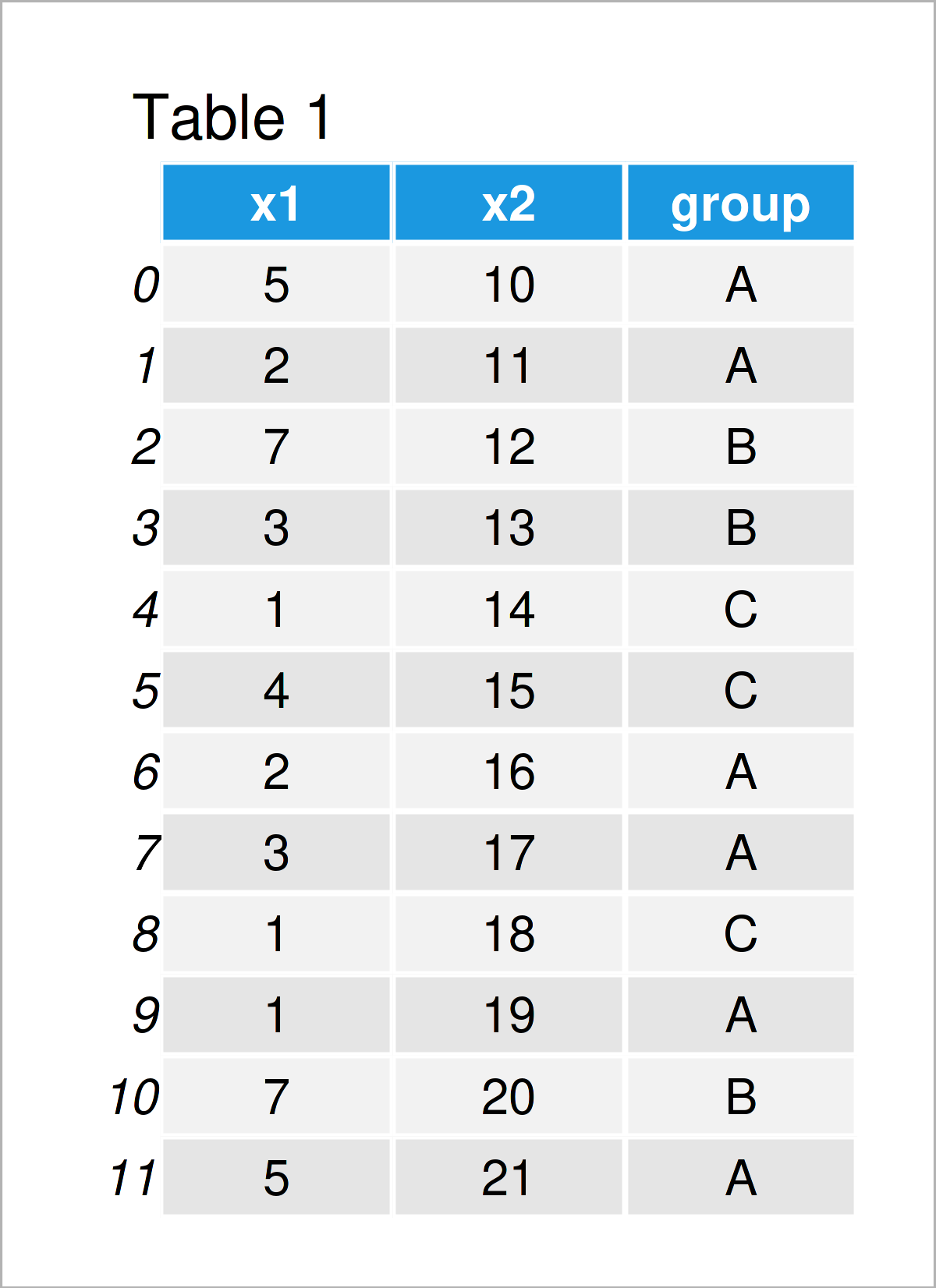# Calculate Sum in Python (5 Examples)

In this article, I’ll demonstrate how to calculate the sum of a list or a pandas DataFrame column in Python programming.

The content of the tutorial looks as follows:

Let’s get started!

## Example 1: Sum of List Object

The following Python code explains how to compute the sum of a list object in Python.

First, we have to create an example list:

```my_list = [1, 4, 3, 9, 1, 3, 5, 7, 3, 2, 1]                      # Create example list
print(my_list)                                                   # Print example list
# [1, 4, 3, 9, 1, 3, 5, 7, 3, 2, 1]```

Also, we have to import the NumPy library to Python:

`import numpy as np                                               # Load NumPy library`

Now, we can apply the sum function of the NumPy library to get the sum of the numbers in our list object:

```print(np.sum(my_list))                                           # Get sum of list
# 39```

The previous output shows our final result, i.e. the sum of all values in our list is 39.

## Example 2: Sum of One Particular Column in pandas DataFrame

The following code demonstrates how to find the sum of one specific pandas DataFrame column.

As a first step, we have to import the pandas library:

`import pandas as pd                                              # Import pandas library to Python`

Next, we have to create an exemplifying pandas DataFrame:

```data = pd.DataFrame({'x1':[5, 2, 7, 3, 1, 4, 2, 3, 1, 1, 7, 5],  # Create pandas DataFrame
'x2':range(10, 22),
'group':['A', 'A', 'B', 'B', 'C', 'C', 'A', 'A', 'C', 'A', 'B', 'A']})
print(data)                                                      # Print pandas DataFrame```In Table 1 it is shown that we have created a pandas DataFrame containing 11 rows and three columns with the previous Python programming code. The variables x1 and x2 are floats and the variable group contains a group indicator.

If we want to calculate the sum of a certain column of our data set (i.e. x1), we can use the following Python code:

```print(data['x1'].sum())                                          # Get sum of one column
# 41```

The sum of the column x1 is 41.

## Example 3: Sum of All Columns in pandas DataFrame

In Example 3, I’ll demonstrate how to return the sum of all columns in a pandas DataFrame.

To achieve this, we can apply the sum function to our entire pandas DataFrame:

```print(data.sum())                                                # Get sum of all columns
# x1                 41
# x2                186
# group    AABBCCAACABA
# dtype: object```

The previous output shows the sum of the float variables x1 and x2 as well as our group indicator as a character string.

## Example 4: Sum of Rows in pandas DataFrame

Until now, we have computed the sums of the columns in our data set. However, this example demonstrates how to get the sums of all rows in a pandas DataFrame.

For this task, we have to specify the axis argument within the sum function to 1.

```print(data.sum(axis = 1))                                        # Get sum of rows
# 0     15
# 1     13
# 2     19
# 3     16
# 4     15
# 5     19
# 6     18
# 7     20
# 8     19
# 9     20
# 10    27
# 11    26
# dtype: int64```

The previous output shows the sum of each row in our data set.

## Example 5: Sum by Group in pandas DataFrame

The following Python programming syntax explains how to print the sum for each group in a pandas DataFrame.

To achieve this result, we have to use the groupby function in combination with the sum function. Within the groupby function, we have to specify the name of our group indicator variable:

```print(data.groupby('group').sum())                               # Get sum by group
#        x1  x2
# group
# A      18  94
# B      17  45
# C       6  47```

As you can see, we have returned the sum of each group in our data set.

## Video, Further Resources & Summary

Would you like to know more about the addition of values in a list or a pandas DataFrame column? Then you might watch the following video on my YouTube channel. I’m showing the Python programming code of this article in the video.

Please accept YouTube cookies to play this video. By accepting you will be accessing content from YouTube, a service provided by an external third party.If you accept this notice, your choice will be saved and the page will refresh.

In addition, you might want to read the other tutorials on my website. I have released several articles that are related to the calculation of the sum of a list or a pandas DataFrame column already.

In this tutorial you have learned how to perform an addition of elements to calculate the sum of a list or a pandas DataFrame column in Python programming. Don’t hesitate to let me know in the comments below, in case you have any further questions. Besides that, don’t forget to subscribe to my email newsletter for regular updates on the newest tutorials.

Subscribe to the Statistics Globe Newsletter